# Dynamical Systems Theory

## 9/26/00 Lecture #4 in Physics 505

###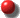Comments on Homework #2 (Bifurcation Diagrams)

• Most everyone did fine
• Number of periodic windows increases with period
• 1 period-3
• 2 period-4
• 3 period-5
• 5 period-6, etc.
• Scaling of number and window width with period is an open question

###Review (last week) - Nonchaotic Multidimensional Flows

• Maps versus flows (discrete versus continuous time)
• Exponential growth or decay (1-D linear)
• Equation:  dx/dt = ax
• Solution:  x = x0eat
• Logistic differential equation (1-D nonlinear)
• Equation: dx/dt = ax(1 - x)
• Equilibrium points at x* = 0 and x* = 1
• Stability determined by sign of df/dx
• Stable equilibrium point is approached asymptotically
• Oscillations and chaos are not possible
• Circular motion (2-D linear)
• Equations:  dx/dt = y,  dy/dt = -x
• Solution:  x = x0 cos ty = -x0 sin t
• Solutions are circles around a center at (0, 0)

•• Center is neutrally stable (neither attracts nor repels)
• Mass on a spring (2-D linear)
• Equations:  dx/dt = v,  dv/dt = -x
• Same as circular motion above
• (x, v) are phase-space variables
• Trajectory forms a phase portrait
• Mass on a spring with friction (dissipative 2-D linear)
• Equations:  dx/dt = v,  dv/dt = -x -bv
• Solution attracts to a stable equilibrium point at (0, 0)
• Nonautonomous systems
• These systems have an explicit time dependence
• Can remove time by defining a new variable
• Dimension increases by 1 (circle becomes a torus)
•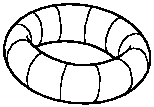• Limit cycles (van der Pol equation)
• Numerical methods for solving ODEs
• Suppose dx/dt = f(x, y) and dy/dt = g(x, y)
• Let h be a small increment of time
• Euler method
• Leap-frog method
• Second order Runge-Kutta
• Fourth order Runge-Kutta

###General 2-D Linear Flows

• Equilibriumx* = 0, y* = 0  (stable)
• Mechanical energy is not conserved (dissipation)
• Best undersood from phase portrait
• Types of equilibrium points in linear 2-D systems:
•  Spiral point (focus)  if b < 2 (underdamped) Radial point (node)  if b > 2 (overdamped) Saddle point (hyperbolic point)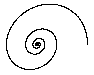• Attractor --> sink,  repellor --> source
• Trajectory cannot intersect itself
• Poincare-Bendixson theorem (no chaos in 2-D)
• Chaos in flows requires at least 3 variables and a nonlinearity

###Stability of Equilibria in 2 Dimensions

• Recall 1-D result:
• dx/dt = f(x)
• Equilibrium: f(x) = 0  ==>  x*
• Stability is determined by sign of df/dx at x*
• Solution near equilibrium is x = x* + (x0 - x*)elt
• Where l = df/dx (at x = x*) is the growth rate
• dx/dt = f(x, y)
• dy/dt = g(x, y)
• Equilibrium pointsf = g = 0  ==> x*, y*
• Calculate the Jacobian matrix J at x*, y*
• Let fx be the partial derivative of f with respect to x, etc.
• The eigenvalues of J are:  (fx - l)(gy - l) = fygx
• This is the characteristic equation (quadratic in 2-D, etc.)
• Solutions are of the form:  x = x0elt, y = y0elt
• There are 2 solutions for (real or complex conjugates)

•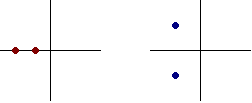###Example: Damped Harmonic Oscillator (2-D linear)

• dx/dt = y  (velocity)
• dy/dt = -x - by  (force)
• fx = 0,  fy = 1,  gx = -1,  gy = -b
• Characteristic equationl2 + bl + 1 = 0
• Solutions: l = -b/2 ± (b2 - 4)1/2/2  (eigenvalues)
• First case:  b > 2
• Overdamped
• Two negative real eigenvalues
• This gives a radial point (node)
• Second case:  b = 2
• Critical damping
• Two negative equal eigenvalues
• Third case:  0 < b < 2
• Underdamped
• Two negative complex eigenvalues
• This gives a spiral point (focus)
• Fourth case:  b < 0  (negative damping)
• Exponential growth
• Two positive eigenvalues
• Attractors become repellors

###Saddle Points (or hyperbolic points)

• Example (note similarity to harmonic oscillator)
• dx/dt = y
• dy/dt = x
• Eigenvalues are l1 = 1, l2 = -1

•• The flow is of the form:

•• Unstable manifold (outset) l1 > 0
• Stable manifold (inset) l2 < 0
• Also called separatrices (trajectories can't cross)
• Separatrices given by the eigenvectors of JR = lR
• Consult any book on linear algebra
• We won't be using the eigenvectors
• The separatrices organize the phase space
• The eigenvalues allow prediction of bifurcations

###Area Contraction (or expansion) in 2-D

• l1l2 = det J = fxgy - fygx  (determinant of J)
• l1 + l2 = trace J = fx + gy  (trace of J)
• Expanding direction:  dE/dt = l1E  (l1 > 0)
• Contracting direction:  dC/dt = l2C (l2 < 0)

•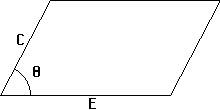• Phase space areaA = CE sin q
• dA/dt = CdE/dt sin q + EdC/dt sin q = CE(l1 + l2) sin q
• dA/dt / A = l1 + l2  (fractional rate of expansion)
• In higher dimension:  dV/dt / V = l1 + l2 + ...  (sum of eigenvalues)
• V is the phase-space volume of initial conditions
• Sum of the eigenvalues must be negative for an attractor

###Flows in 3 Dimensions

• Types of equilibria
• Cubic characteristic equation
• Three eigenvalues (3 real or 1 real)
• Attracting equilibrium points (2 types)

•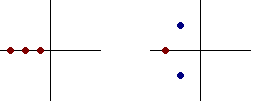• Repelling equilibrium points (2 types)

•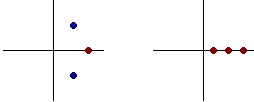•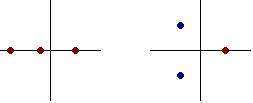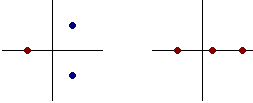• Index:  number of eigenvalues with Re(l) > 0

• [or dimension of the unstable manifold]
• Chaos occurs with 2 or more unstable equilibria
• Attractors in 3-D flows
• Equilibrium point (as in 1 and 2 dimensions)
• Limit cycle (as in 2 dimensions)
• Torus (quasiperiodic - 2 incommensurate frequencies)
• Strange (chaotic) attractors
• Examples of chaotic dissipative flows in 3-D:

J. C. Sprott | Physics 505 Home Page | Previous Lecture | Next Lecture